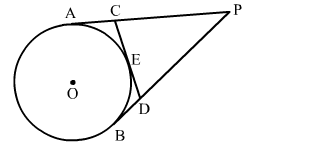# From an external point P, tangents PA and PB are drawn to a circle with centre O.

Question:

From an external point P, tangents PA and PB are drawn to a circle with centre O. If CD is the tangent to the circle at a point E and PA = 14 cm, find the perimeter of ΔPCD.Solution:

Given, $\mathrm{PA}$ and $\mathrm{PB}$ are the tangents to a circle with centre $\mathrm{O}$ and $\mathrm{CD}$ is a tangent at $\mathrm{E}$ and $\mathrm{PA}=14 \mathrm{~cm} .$

Tangents drawn from an external point are equal.

$\therefore P A=P B, C A=C E$ and $D B=D E$

Perimeter of $\triangle P C D=P C+C D+P D$

$=(P A-C A)+(C E+D E)+(P B-D B)$

$=(P A-C E)+(C E+D E)+(P B-D E)$

$=(P A+P B)$

$=2 \mathrm{PA} \quad(\because \mathrm{PA}=\mathrm{PB})$

$=(2 \times 14) \mathrm{cm}$

$=28 \mathrm{~cm}$

$\therefore$ Perimeter of $\triangle P C D=28 \mathrm{~cm}$.